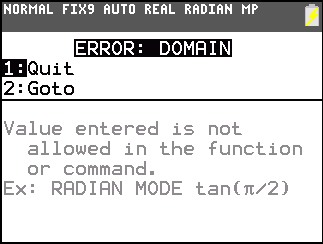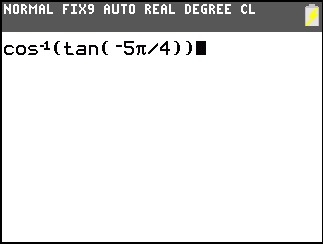# Knowledge Base

## Solution 34616: Inverse Cosine of the Tangent of -5p/4 on the TI-83 Plus and TI-84 Plus Family of Graphing Calculators.

### Why is the inverse cosine of tan(-5pi/4) returning a Domain Error message on the TI-83 Plus and TI-84 Plus family of graphing calculators?

This is a classic problem with floating point arithmetic that is used in calculators and almost all computers.

The main problem is tan(-5*π/4). The TI-83 Plus and TI-84 Plus family of graphing calculators only perform calculations with 14 digits in the memory, the calculation of tan (-5*π/4) loses some accuracy on the last digit. Internally, the answer of tan(-5*π/4) is not -1 but something like -1.0000000000001. The display routine on the calculator is designed to display -1 instead of the internal number. However, arcos function will take the internal number -1.0000000000001 as input and determines it is out-of-range.

Try the following example to get the same result:

1) Press [tan] [ (-) ]  [2nd] [^] [/]  [ ) ] [sto→] [alpha] [math] [enter] to store the result tan(-5*π/4) to the variable A
2) Press [2nd] [cos] [alpha] [math] [ ) ] [enter] to calculate inverse cos(A) which yields the same error as cos^-1(tan(-5*π/4)).Please Note: The inverse cos function is displayed as follows:Degree mode will work because (-225) is an integer. The same kind of problem exists if the input is a general floating point.

Please see the TI-83 Plus and TI-84 Plus Family guidebooks for additional information.

Last updated: 7/18/2023